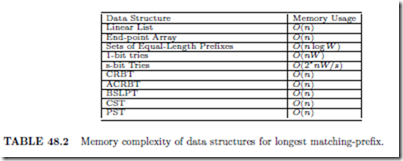# IP Router Tables:Introduction and Longest Matching-Preﬁx

## Introduction

In the d-dimensional packet classiﬁcation problem, each rule has a d-ﬁeld ﬁlter. In this chapter, we are concerned solely with 1-dimensional packet classiﬁcation. It should be noted, that data structures for multidimensional packet classiﬁcation are usually built on top of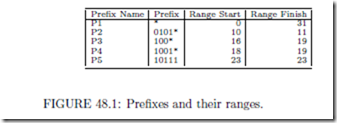data structures for 1-dimensional packet classiﬁcation. Therefore, the study of data structures for 1-dimensional packet classiﬁcation is fundamental to the design and development of data structures for d-dimensional, d > 1, packet classiﬁcation.

For the 1-dimensional packet classiﬁcation problem, we assume that the single ﬁeld in the ﬁlter is the destination ﬁeld and that the action is the next hop for the packet. With these assumptions, 1-dimensional packet classiﬁcation is equivalent to the destination-based packet forwarding problem. Henceforth, we shall use the terms rule table and router table to mean tables in which the ﬁlters have a single ﬁeld, which is the destination address. This single ﬁeld of a ﬁlter may be speciﬁed in one of two ways:

1. As a range. For example, the range [35, 2096] matches all destination addresses d such that 35 d 2096.

2. As an address/mask pair. Let xi denote the ith bit of x. The address/mask pair a/m matches all destination addresses d for which di = ai for all i for which mi = 1. That is, a 1 in the mask speciﬁes a bit position in which d and a must agree, while a 0 in the mask speciﬁes a don’t care bit position. For example, the address/mask pair 101100/011101 matches the destination addresses 101100, 101110, 001100, and 001110.

When all the 1-bits of a mask are to the left of all 0-bits, the address/mask pair speciﬁes an address preﬁx. For example, 101100/110000 matches all destination addresses that have the preﬁx 10 (i.e., all destination addresses that begin with 10). In this case, the address/mask pair is simply represented as the preﬁx 10*, where the * denotes a sequence of don’t care bits. If W is the length, in bits, of a destination address, then the * in 10* represents all sequences of W 2 bits.

In IPv4 the address and mask are both 32 bits, while in IPv6 both of these are 128 bits.

Notice that every preﬁx may be represented as a range. For example, when W = 6, the preﬁx 10* is equivalent to the range [32, 47]. A range that may be speciﬁed as a preﬁx for some W is called a prex range. The speciﬁcation 101100/011101 may be abbreviated to ?011?0, where ? denotes a don’t-care bit. This speciﬁcation is not equivalent to any single range. Also, the range speciﬁcation [3,6] isn’t equivalent to any single address/mask speciﬁcation.

Figure 48.1 shows a set of ﬁve preﬁxes together with the start and ﬁnish of the range for each. This ﬁgure assumes that W = 5. The preﬁx P1 = *, which matches all legal destination addresses, is called the default preﬁx.

Suppose that our router table is comprised of ﬁve rules R1–R5 and that the ﬁlters for these ﬁve rules are P1–P5, respectively. Let N1–N5, respectively, be the next hops for these ﬁve rules. The destination address 18 is matched by rules R1, R3, and R5 (equivalently, by preﬁxes P1, P3, and P5). So, N1, N3, and N5 are candidates for the next hop for incoming packets that are destined for address 18. Which of the matching rules (and associated action) should be selected? When more than one rule matches an incoming packet, a tie occurs. To select one of the many rules that may match an incoming packet, we use a tie breaker.

Let RS be the set of rules in a rule table and let FS be the set of ﬁlters associated with these rules. rules(d, RS) (or simply rules(d) when RS is implicit) is the subset of rules of RS that match/cover the destination address d. f ilters(d, F S) and f ilters(d) are deﬁned

similarly. A tie occurs whenever |rules(d)| > 1 (equivalently, |f ilters(d)| > 1).

Three popular tie breakers are:

1. First matching rule in table. The rule table is assumed to be a linear list () of rules with the rules indexed 1 through n for an n-rule table. The action corresponding to the ﬁrst rule in the table that matches the incoming packet is used. In other words, for packets with destination address d, the rule of rules(d) that has least index is selected.

For our example router table corresponding to the ﬁve preﬁxes of Figure 48.1, rule R1 is selected for every incoming packet, because P1 matches every destination address. When using the ﬁrst-matching-rule criteria, we must index the rules carefully. In our example, P1 should correspond to the last rule so that every other rule has a chance to be selected for at least one destination address.

2. Highest-priority rule. Each rule in the rule table is assigned a priority. From among the rules that match an incoming packet, the rule that has highest priority wins is selected. To avoid the possibility of a further tie, rules are assigned diﬀerent priorities (it is actually suﬃcient to ensure that for every destination address d, rules(d) does not have two or more highest-priority rules).

Notice that the ﬁrst-matching-rule criteria is a special case of the highest-priority criteria (simply assign each rule a priority equal to the negative of its index in the linear list).

3. Most-speciﬁc-rule matching. The ﬁlter F 1 is more speciﬁc than the ﬁlter F 2 iﬀ F 2 matches all packets matched by F 1 plus at least one additional packet. So, for example, the range [2, 4] is more speciﬁc than [1, 6], and [5, 9] is more speciﬁc than [5, 12]. Since [2, 4] and [8, 14] are disjoint (i.e., they have no address in common), neither is more speciﬁc than the other. Also, since [4, 14] and [6, 20] intersect, neither is more speciﬁc than the other. The preﬁx 110* is more speciﬁc than the preﬁx 11*.

In most-speciﬁc-rule matching, ties are broken by selecting the matching rule that has the most speciﬁc ﬁlter. When the ﬁlters are destination preﬁxes, the most-speciﬁc-rule that matches a given destination d is the longestpreﬁx in f ilters(d). Hence, for preﬁx ﬁlters, the most-speciﬁc-rule tie breaker is equivalent to the longest-matching-preﬁx criteria used in router tables. For our example rule set, when the destination address is 18, the longest matching-preﬁx is P4. When the ﬁlters are ranges, the most-speciﬁc-rule tie breaker requires us to se- lect the most speciﬁc range in f ilters(d). Notice also that most-speciﬁc-range matching is a special case of the the highest-priority rule. For example, when the ﬁlters are preﬁxes, set the preﬁx priority equal to the preﬁx length. For the case of ranges, the range priority equals the negative of the range size.

In a static rule table, the rule set does not vary in time. For these tables, we are concerned primarily with the following metrics:

1. Time required to process an incoming packet. This is the time required to search the rule table for the rule to use.

2. Preprocessing time. This is the time to create the rule-table data structure.

3. Storage requirement. That is, how much memory is required by the rule-table data structure?

In practice, rule tables are seldom truly static. At best, rules may be added to or deleted from the rule table infrequently. Typically, in a “static” rule table, inserts/deletes are batched and the rule-table data structure reconstructed as needed.

In a dynamic rule table, rules are added/deleted with some frequency. For such tables, inserts/deletes are not batched. Rather, they are performed in real time. For such tables, we are concerned additionally with the time required to insert/delete a rule. For a dynamic rule table, the initial rule-table data structure is constructed by starting with an empty data structures and then inserting the initial set of rules into the data structure one by one. So, typically, in the case of dynamic tables, the preprocessing metric, mentioned above, is very closely related to the insert time.

In this paper, we focus on data structures for static and dynamic router tables (1- dimensional packet classiﬁcation) in which the ﬁlters are either preﬁxes or ranges.

## Longest Matching-Preﬁx

Linear List

In this data structure, the rules of the rule table are stored as a linear list () L. Let LM P (d) be the longest matching-preﬁx for address d. LM P (d) is determined by examining the preﬁxes in L from left to right; for each preﬁx, we determine whether or not that preﬁx matches d; and from the set of matching preﬁxes, the one with longest length is selected. To insert a rule q, we ﬁrst search the list L from left to right to ensure that L doesn’t already have a rule with the same ﬁlter as does q. Having veriﬁed this, the new rule q is added to the end of the list. Deletion is similar. The time for each of the operations determine LM P (d), insert a rule, delete a rule is O(n), where n is the number of rules in L. The memory required is also O(n).

Note that this data structure may be used regardless of the form of the ﬁlter (i.e., ranges, Boolean expressions, etc.) and regardless of the tie breaker in use. The time and memory complexities are unchanged.

Although the linear list is not a suitable data structure for a purely software implementation of a router table with a large number of preﬁxes, it is leads to a very practical solution using TCAMs (ternary content-addressable memories) [28, 34]. Each memory cell of a TCAM may be set to one of three states 0, 1, and don’t care. The preﬁxes of a router table are stored in a TCAM in descending order of preﬁx length. Assume that each word of the TCAM has 32 cells. The preﬁx 10* is stored in a TCAM word as 10???…?,  where? denotes a don’t care and there are 30 ?s in the given sequence. To do a longest-preﬁx match, the destination address is matched, in parallel, against every TCAM entry and the ﬁrst (i.e., longest) matching entry reported by the TCAM arbitration logic. So, using a TCAM and a sorted-by-length linear list, the longest matching-preﬁx can be determined in O(1) time. A preﬁx may be inserted or deleted in O(W ) time, where W is the length of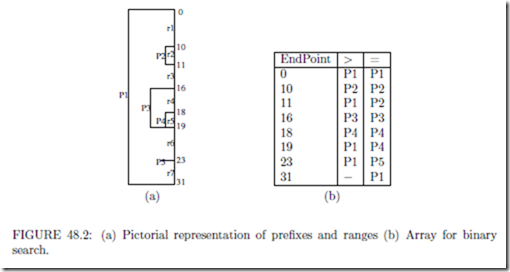the longest preﬁx §. For example, an insert of a preﬁx of length 3 (say) can be done by relocating a the ﬁrst preﬁx of length 1 to the end of the list; ﬁlling the vacated slot with the ﬁrst preﬁx of length 2; and ﬁnally ﬁlling this newly vacated spot with the preﬁx of length 3 that is to be inserted.

Despite the simplicity and eﬃciency of using TCAMs, TCAMs present problems in real applications . For example, TCAMs consume a lot of power and board area.

End-Point Array

Lampson, Srinivasan, and Varghese  have proposed a data structure in which the end points of the ranges deﬁned by the preﬁxes are stored in ascending order in an array. LM P (d) is found by performing a binary search on this ordered array of end points.

Preﬁxes and their ranges may be drawn as nested rectangles as in Figure 48.2(a), which gives the pictorial representation of the ﬁve preﬁxes of Figure 48.1.

In the data structure of Lampson et al. , the distinct range end-points are stored in ascending order as in Figure 48.2(b). The distinct end-points (range start and ﬁnish points) for the preﬁxes of Figure 48.1 are [0, 10, 11, 16, 18, 19, 23, 31]. Let ri, 1 i q 2n be the distinct range end-points for a set of n preﬁxes. Let rq+1 = . With each distinct range end-point, ri, 1 i q, the array stores LM P (d) for d such that (a) ri < d < ri+1 (this is the column labeled “>” in Figure 48.2(b)) and (b) ri = d (column labeled “=”). Now, LM P (d), r1 d rq can be determined in O(log n) time by performing a binary search to ﬁnd the unique i such that ri d < ri+1. If ri = d, LM P (d) is given by the “=” entry; otherwise, it is given by the “>” entry. For example, since d = 20 satisﬁes 19 d < 23 and since d j= 19, the “>” entry of the end point 19 is used to determine that LM P (20) is P1.

As noted by Lampson et al. , the range end-point table can be built in O(n) time (this assumes that the end points are available in ascending order). Unfortunately, as stated in , updating the range end-point table following the insertion or deletion of a preﬁx also takes O(n) time because O(n) “>” and/or “=” entries may change. Although Lampson et al.  provide ways to reduce the complexity of the search for the LMP by a constant factor, these methods do not result in schemes that permit preﬁx insertion and deletion in O(log n) time.

It should be noted that the end-point array may be used even when ties are broken by selecting the ﬁrst matching rule or the highest-priority matching rule. Further, the method applies to the case when the ﬁlters are arbitrary ranges rather than simply preﬁxes. The complexity of the preprocessing step (i.e., creation of the array of ordered end-points) and the search for the rule to use is unchanged. Further, the memory requirements are the same, O(n) for an n-rule table, regardless of the tie breaker and whether the ﬁlters are preﬁxes or general ranges.

Sets of Equal-Length Preﬁxes

Waldvogel et al.  have proposed a data structure to determine LM P (d) by performing a binary search on preﬁx length. In this data structure, the preﬁxes in the router table T are partitioned into the sets S0, S1, ... such that Si contains all preﬁxes of T whose length is i. For simplicity, we assume that T contains the default preﬁx *. So, S0 = {∗}. Next, each Si is augmented with markers that represent preﬁxes in Sj such that j > i and i is on the binary search path to Sj . For example, suppose that the length of the longest preﬁx of T is 32 and that the length of LM P (d) is 22. To ﬁnd LM P (d) by a binary search on length, we will ﬁrst search S16 for an entry that matches the ﬁrst 16 bits of d. This searchwill need to be successful for us to proceed to a larger length. The next search will be in S24. This search will need to fail. Then, we will search S20 followed by S22. So, the path followed by a binary search on length to get to S22 is S16, S24, S20, and S22. For this to be followed, the searches in S16, S20, and S22 must succeed while that in S24 must fail. Since the length of LM P (d) is 22, T has no matching preﬁx whose length is more than 22. So, the search in S24 is guaranteed to fail. Similarly, the search in S22 is guaranteed to succeed. However, the searches in S16 and S20 will succeed iﬀ T has matching preﬁxes of length 16 and 20. To ensure success, every length 22 preﬁx P places a marker in S16 and S20, the marker in S16 is the ﬁrst 16 bits of P and that in S20 is the ﬁrst 20 bits in P . Note that a marker M is placed in Si only if Si doesn’t contain a preﬁx equal to M . Notice also that for each i, the binary search path to Si has O(log lmax) = O(log W ), where lmax is the length of the longest preﬁx in T , Sj s on it. So, each preﬁx creates O(log W ) markers. With each marker M in Si, we record the longest preﬁx of T that matches M (the length of this longest matching-preﬁx is necessarily smaller than i).

To determine LM P (d), we begin by setting lef tEnd = 0 and rightEnd = lmax. The repetitive step of the binary search requires us to search for an entry in Sm, where m = l(lef tEnd + rightEnd)/2J, that equals the ﬁrst m bits of d. If Sm does not have such an entry, set right End = m 1. Otherwise, if the matching entry is the preﬁx P , P becomes the longest matching-preﬁx found so far. If the matching entry is the marker M , the preﬁx recorded with M is the longest matching-preﬁx found so far. In either case, set lef tEnd = m + 1. The binary search terminates when lef tEnd > rightEnd.

One may easily establish the correctness of the described binary search. Since, each preﬁx creates O(log W ) markers, the memory requirement of the scheme is O(n log W ). When each set Si is represented as a hash table, the data structure is called SELPH (sets of equal length preﬁxes using hash tables). The expected time to ﬁnd LM P (d) is O(log W ) when the router table is represented as an SELPH. When inserting a preﬁx, O(log W ) markers must also be inserted. With each marker, we must record a longest-matching preﬁx. The expected time to ﬁnd these longest matching-preﬁxes is O(log2 W ). In addition, we may need to update the longest-matching preﬁx information stored with the O(n log W ) markers at lengths greater than the length of the newly inserted preﬁx. This takes O(n log2 W ) time. So, the expected insert time is O(n log2 W ). When deleting a preﬁx P , we must search all hash tables for markers M that have P recorded with them and then update the recorded preﬁx for each of these markers. For hash tables with a bounded loading density, the expected time for a delete (including marker-preﬁx updates) is O(n log2 W ). Waldvogel et al.  have shown that by inserting the preﬁxes in ascending order of length, an n-preﬁx SELPH may be constructed in O(n log2 W ) time.

When each set is represented as a balanced search tree (see Chapter 10), the data structure is called SELPT. In an SELPT, the time to ﬁnd LM P (d) is O(log n log W ); the insert time is O(n log n log2 W ); the delete time is O(n log n log2 W ); and the time to construct the data structure for n preﬁxes is O(W + n log n log2 W ).

In the full version of , Waldvogel et al. show that by using a technique called marker partitioning, the SELPH data structure may be modiﬁed to have a search time of O(α + log W ) and an insert/delete time of O(α α nW log W ), for any α > 1.

Because of the excessive insert and delete times, the sets of equal-length preﬁxes data structure is suitable only for static router tables. Note that in an actual implementation of SELPH or SELPT, we need only keep the non-empty Sis and do a binary search over the collection of non-empty Sis. Srinivasan and Varghese  have proposed the use of controlled preﬁx-expansion to reduce the number of non-empty sets Si. The details of their algorithm to reduce the number of lengths are given in . The complexity of their algorithm is O(nW 2), where n is the number of preﬁxes, and W is the length of the longest preﬁx. The algorithm of  does not minimize the storage required by the preﬁxes and markers for the resulting set of preﬁxes. Kim and Sahni  have developed an algorithm that minimizes storage requirement but takes O(nW 3 + kW 4) time, where k is the desired number of non-empty Sis. Additionally, Kim and Sahni  propose improvements to the heuristic of .

We note that Waldvogel’s scheme is very similar to the k-ary search-on-length scheme de- veloped by Berg et al.  and the binary search-on-length schemes developed by Willard . Berg et al.  use a variant of stratiﬁed trees  for one-dimensional point location in a set of n disjoint ranges. Willard  modiﬁed stratiﬁed trees and proposed the y-fast trie data structure to search a set of disjoint ranges. By decomposing ﬁlter ranges that are not disjoint into disjoint ranges, the schemes of [4, 33] may be used for longest-preﬁx matching in static router tables. The asymptotic complexity for a search using the schemes of [4, 33] is the same as that of Waldvogel’s scheme. The decomposition of overlapping ranges into disjoint ranges is feasible for static router tables but not for dynamic router tables because a large range may be decomposed into O(n) disjoint small ranges.

Tries

1- Bit Tries

A 1-bit trie is a tree-like structure in which each node has a left child, left data, right child, and right data ﬁeld. Nodes at levelll l 1 of the trie store preﬁxes whose length is l. If the rightmost bit in a preﬁx whose length is l is 0, the preﬁx is stored in the left data ﬁeld of a node that is at level l 1; otherwise, the preﬁx is stored in the right data ﬁeld of a node that is at level l 1. At level i of a trie, branching is done by examining bit i (bits are numbered from left to right beginning with the number 0) of a preﬁx or destination address. When bit i is 0, we move into the left subtree; when the bit is 1, we move into the right subtree. Figure 48.3(a) gives the preﬁxes in the 8-preﬁx example of , and Figure 48.3(b) shows the corresponding 1-bit trie. The preﬁxes in Figure 48.3(a) are numbered and ordered as in .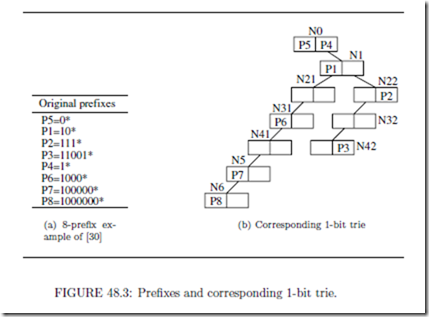The 1-bit tries described here are an extension of the 1-bit tries described in . The primary diﬀerence being that the 1-bit tries of  are for the case when no key is a preﬁx of another. Since in router-table applications, this condition isn’t satisﬁed, the 1-bit trie representation of  is extended so that keys of length l are stored in nodes at level l 1 of the trie. Note that at most two keys may be stored in a node; one of these has bit l equal to 0 and other has this bit equal to 1. In this extension, every node of the 1-bit trie has 2 child pointers and 2 data ﬁelds. The height of a 1-bit trie is O(W ).

For any destination address d, all preﬁxes that match d lie on the search path determined by the bits of d. By following this search path, we may determine the longest matching- preﬁx, the ﬁrst preﬁx in the table that matches d, as well as the highest-priority matching- preﬁx in O(W ) time. Further, preﬁxes may be inserted/deleted in O(W ) time. The memory required by the 1-bit trie is O(nW ).

IPv4 backbone routers may have more than 100 thousand preﬁxes. Even though the preﬁxes in a backbone router may have any length between 0 and W , there is a concentration of preﬁxes at lengths 16 and 24, because in the early days of the Internet, Internet address assignment was done by classes. All addresses in a class B network have the same ﬁrst 16 bits, while addresses in the same class C network agree on the ﬁrst 24 bits. Addresses in class A networks agree on their ﬁrst 8 bits. However, there can be at most 256 class A networks (equivalently, there can be at most 256 8-bit preﬁxes in a router table). For our backbone routers that occur in practice , the number of nodes in a 1-bit trie is between 2n and 3n. Hence, in practice, the memory required by the 1-bit-trie representation is O(n).

Fixed-Stride Tries

Since the trie of Figure 48.3(b) has a height of 6, a search into this trie may make up to 7 memory accesses, one access for each node on the path from the root to a node at level 6 of the trie. The total memory required for the 1-bit trie of Figure 48.3(b) is 20 units (each node requires 2 units, one for each pair of (child, data) ﬁelds).

When 1-bit tries are used to represent IPv4 router tables, the trie height may be as much as 31. A lookup in such a trie takes up to 32 memory accesses.

Degermark et al.  and Srinivasan and Varghese  have proposed the use of ﬁxed- stride tries to enable fast identiﬁcation of the longest matching preﬁx in a router table. The stride of a node is deﬁned to be the number of bits used at that node to determine which branch to take. A node whose stride is s has 2s child ﬁelds (corresponding to the 2s possible values for the s bits that are used) and 2s data ﬁelds. Such a node requires 2s memory units. In a ﬁxed-stride trie (FST), all nodes at the same level have the same stride; nodes at diﬀerent levels may have diﬀerent strides.

Suppose we wish to represent the preﬁxes of Figure 48.3(a) using an FST that has three levels. Assume that the strides are 2, 3, and 2. The root of the trie stores preﬁxes whose length is 2; the level one nodes store preﬁxes whose length is 5 (2 + 3); and level three nodes store preﬁxes whose length is 7 (2 + 3 + 2). This poses a problem for the preﬁxes of our example, because the length of some of these preﬁxes is diﬀerent from the storeable lengths. For instance, the length of P5 is 1. To get around this problem, a preﬁx with a nonpermissible length is expanded to the next permissible length. For example, P5 = 0* is expanded to P5a = 00* and P5b = 01*. If one of the newly created preﬁxes is a duplicate, natural dominance rules are used to eliminate all but one occurrence of the preﬁx. For instance, P4 = 1* is expanded to P4a = 10* and P4b = 11*. However, P1 = 10* is to be chosen over P4a = 10*, because P1 is a longer match than P4. So, P4a is eliminated. Because of the elimination of duplicate preﬁxes from the expanded preﬁx set, all preﬁxes are distinct.

Figure 48.4(a) shows the preﬁxes that result when we expand the preﬁxes of Figure 48.3 to lengths 2, 5, and 7. Figure 48.4(b) shows the corresponding FST whose height is 2 and whose strides are 2, 3, and 2.

Since the trie of Figure 48.4(b) can be searched with at most 3 memory references, it represents a time performance improvement over the 1-bit trie of Figure 48.3(b), which requires up to 7 memory references to perform a search. However, the space requirements of the FST of Figure 48.4(b) are more than that of the corresponding 1-bit trie. For the root of the FST, we need 8 ﬁelds or 4 units; the two level 1 nodes require 8 units each; and the level 3 node requires 4 units. The total is 24 memory units.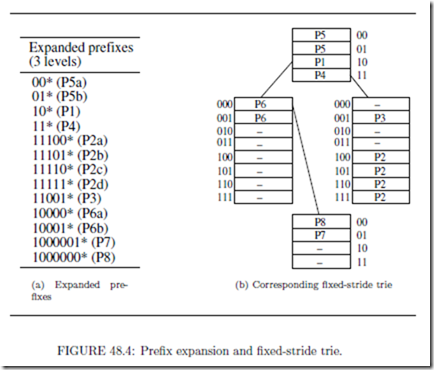We may represent the preﬁxes of Figure 48.3(a) using a one-level trie whose root has a stride of 7. Using such a trie, searches could be performed making a single memory access. However, the one-level trie would require 27 = 128 memory units.

For IPv4 preﬁx sets, Degermark et al.  propose the use of a three-level trie in which the strides are 16, 8, and 8. They propose encoding the nodes in this trie using bit vectors to reduce memory requirements. The resulting data structure requires at most 12 memory accesses. However, inserts and deletes are quite expensive. For example, the insertion of the preﬁx 1* changes up to 215 entries in the trie’s root node. All of these changes may propagate into the compacted storage scheme of .

Lampson et al.  have proposed the use of hybrid data structures comprised of a stride- 16 root and an auxiliary data structure for each of the subtries of the stride-16 root. This auxiliary data structure could be the end-point array of Section 48.2.2 (since each subtrie is expected to contain only a small number of preﬁxes, the number of end points in each end-point array is also expected to be quite small). An alternative auxiliary data structure suggested by Lampson et al.  is a 6-way search tree for IPv4 router tables. In the case of these 6-way trees, the keys are the remaining up to 16 bits of the preﬁx (recall that the stride-16 root consumes the ﬁrst 16 bits of a preﬁx). For IPv6 preﬁxes, a multicolumn scheme is suggested . None of these proposed structures is suitable for a dynamic table. In the ﬁxed-stride trie optimization (FSTO) problem, we are given a set P of preﬁxes and an integer k. We are to select the strides for a k-level FST in such a manner that the k-level FST for the given preﬁxes uses the smallest amount of memory.

For some P , a k-level FST may actually require more space than a (k 1)-level FST. For example, when P = {00*, 01*, 10*, 11*}, the unique 1-level FST for P requires 4 memory units while the unique 2-level FST (which is actually the 1-bit trie for P ) requires 6 memory units. Since the search time for a (k 1)-level FST is less than that for a k-level tree, we would actually prefer (k 1)-level FSTs that take less (or even equal) memory over k-level FSTs. Therefore, in practice, we are really interested in determining the best FST that uses at most k levels (rather than exactly k levels). The modiﬁed MSTO problem (MFSTO) is to determine the best FST that uses at most k levels for the given preﬁx set P .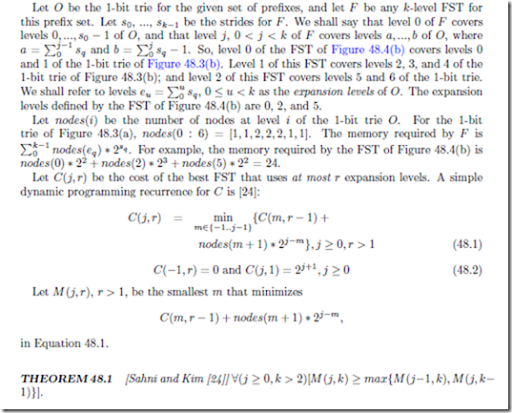Theorem 48.1 results in an algorithm to compute C(W 1, k) in O(kW 2). Using the computed M values, the strides for the OFST that uses at most k expansion levels may be determined in an additional O(k) time. Although the resulting algorithm has the same asymptotic complexity as does the optimization algorithm of Srinivasan and Varghese , experiments conducted by Sahni and Kim  using real IPv4 preﬁx-data-sets indicate that the algorithm based on Theorem 48.1 runs 2 to 4 times as fast.

Basu and Narliker  consider implementing FST router tables on a pipelined architecture. Each level of the FST is assigned to a unique pipeline stage. The optimization problem to be solved in this application requires an FST that has a number of levels no more than the number of pipeline stages, the memory required per level should not exceed the available per stage memory, and the total memory required is minimum subject to the stated constraints.

Variable-Stride Tries

In a variable-stride trie (VST) , nodes at the same level may have diﬀerent strides.

Figure 48.5 shows a two-level VST for the 1-bit trie of Figure 48.3. The stride for the root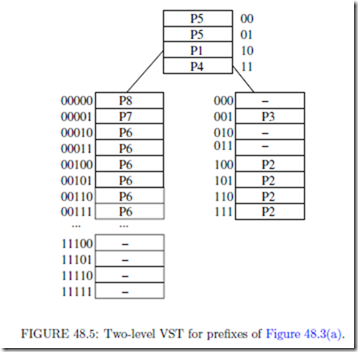is 2; that for the left child of the root is 5; and that for the root’s right child is 3. The memory requirement of this VST is 4 (root) + 32 (left child of root) + 8 (right child of root) = 44.

Since FSTs are a special case of VSTs, the memory required by the best VST for a given preﬁx set P and number of expansion levels k is less than or equal to that required by the best FST for P and k. Despite this, FSTs may be preferred in certain router applications “because of their simplicity and slightly faster search time” .

Let r-VST be a VST that has at most r levels. Let Opt(N, r) be the cost (i.e., memory requirement) of the best r-VST for a 1-bit trie whose root is N . Nilsson and Karlsson  propose a greedy heuristic to construct optimal VSTs. The resulting VSTs are known as LC-tries (level-compressed tries) and were ﬁrst proposed in a more general context by Andersson and Nilsson . An LC-tries obtained from a 1-bit trie by replacing full subtries of the 1-bit trie by single multibit nodes. This replacement is done by examining the 1-bit trie top to bottom (i.e., from root to leaves). Srinivasan and Varghese , have obtained the following dynamic programming recurrence for Opt(N, r).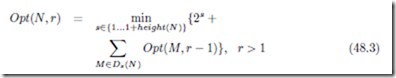where Ds(N ) is the set of all descendants of N that are at level s of N . For example, D1(N ) is the set of children of N and D2(N ) is the set of grandchildren of N . height(N ) is the maximum level at which the trie rooted at N has a node. For example, in Figure 48.3(b), the height of the trie rooted at N1 is 5. When r = 1,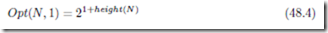Srinivasan and Varghese , describe a way to determine Opt(R, k) using Equations 48.3 and 48.4. The complexity of their algorithm is O(p W k), where p is the number of nodes in the 1-bit trie for the preﬁxes (p = O(n) for realistic router tables). Sahni and Kim  provide an alternative way to compute Opt(R, k) in O(pW k) time. The algorithm of , however, performs fewer operations and has fewer cache misses. When the cost of operations dominates the run time, the algorithm of  is expected to be about 6 times as fast as that of  (for available router databases). When cache miss time dominates the run time, the algorithm of  could be 12 times as fast when k = 2 and 42 times as fast when k = 7.

We describe the formulation used in . Let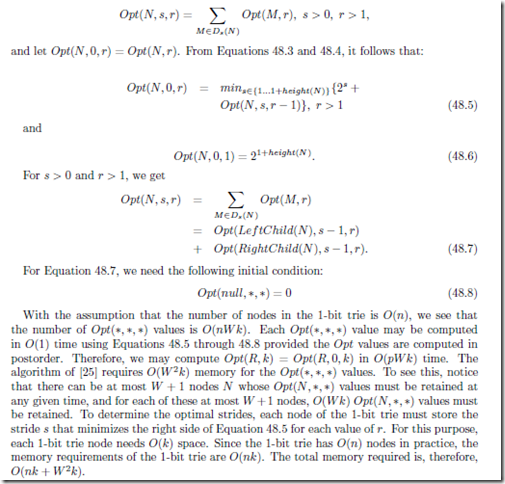In practice, we may prefer an implementation that uses considerably more memory. If we associate a cost array with each of the p nodes of the 1-bit trie, the memory requirement increases to O(pW k). The advantage of this increased memory implementation is that the optimal strides can be recomputed in O(W 2k) time (rather than O(pW k)) following each insert or delete of a preﬁx. This is so because, the Opt(N, , ) values need be recomputed only for nodes along the insert/delete path of the 1-bit trie. There are O(W ) such nodes.

Faster algorithms to determine optimal 2- and 3-VSTs also are developed in .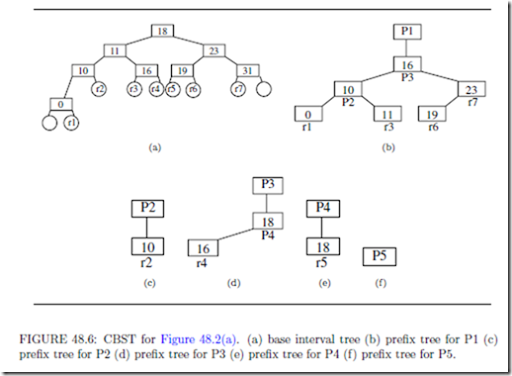Binary Search Trees

Sahni and Kim  propose the use of a collection of red-black trees (see Chapter 10) to determine LM P (d). The CRBT comprises a front-end data structure that is called the binary interval tree (BIT) and a back-end data structure called a collection of preﬁx trees (CPT). For any destination address d, deﬁne the matching basic interval to be a basic interval with the property that ri d ri+1 (note that some ds have two matching basic intervals).

The BIT is a binary search tree that is used to search for a matching basic interval for d. The BIT comprises internal and external nodes and there is one internal node for each ri. Since the BIT has q internal nodes, it has q + 1 external nodes. The ﬁrst and last of these, in inorder, have no signiﬁcance. The remaining q 1 external nodes, in inorder, represent the q 1 basic intervals of the given preﬁx set. Figure 48.6(a) gives a possible (we say possible because, any red-black binary search tree organization for the internal nodes will suﬃce) BIT for our ﬁve-preﬁx example of Figure 48.2(a).

Internal nodes are shown as rectangles while circles denote external nodes. Every external node has three pointers: startPointer, ﬁnishPointer, and basicIntervalPointer. For an external node that represents the basic interval [ri, ri+1 ], startPointer (ﬁnishPointer) points to the header node of the preﬁx tree (in the back-end structure) for the preﬁx (if any) whose range start and ﬁnish points are ri (ri+1). Note that only preﬁxes whose length is W can have this property. basicIntervalPointer points to a preﬁx node in a preﬁx tree of the back-end structure. In Figure 48.6(a), the labels in the external (circular) nodes identify the represented basic interval. The external node with r1 in it, for example, has a basicIntervalPointer to the rectangular node labeled r1 in the preﬁx tree of Figure 48.6(b).

For each preﬁx and basic interval, x, deﬁne next(x) to be the smallest range preﬁx (i.e., the longest preﬁx) whose range includes the range of x. For the example of Figure 48.2(a), the next() values for the basic intervals r1 through r7 are, respectively, P1, P2, P1, P3, P4, P1, and P1. Notice that the next value for the range [ri, ri+1] is the same as the “>” value for ri in Figure 48.2(b), 1 i< q. The next() values for the nontrivial preﬁxes P1 through P4 of Figure 48.2(a) are, respectively, “-”, P1, P1, and P3.

The back-end structure, which is a collection of preﬁx trees (CPT), has one preﬁx tree for each of the preﬁxes in the router table. Each preﬁx tree is a red-black tree. The preﬁx tree for preﬁx P comprises a header node plus one node, called a prex node, for every nontrivial preﬁx or basic interval x such that next(x) = P . The header node identiﬁes the preﬁx P for which this is the preﬁx tree. The preﬁx trees for each of the ﬁve preﬁxes of Figure 48.2(a)

are shown in Figures 48.6(b)-(f).

Notice that preﬁx trees do not have external nodes and that the preﬁx nodes of a preﬁx tree store the start point of the range or preﬁx represented by that preﬁx node. In the ﬁgures, the start points of the basic intervals and preﬁxes are shown inside the preﬁx nodes while the basic interval or preﬁx name is shown outside the node.

The search for LM P (d) begins with a search of the BIT for the matching basic interval for d. Suppose that external node Q of the BIT represents this matching basic interval.

When the destination address equals the left (right) end-point of the matching basic in- terval and startPointer (ﬁnishPointer) is not null, LM P (d) is pointed to by startPointer (ﬁnishPointer). Otherwise, the back-end CPT is searched for LM P (d). The search of the back-end structure begins at the node Q.basicIntervalP ointer. By following parent pointers from Q.basicIntervalP ointer, we reach the header node of the preﬁx tree that corresponds to LM P (d).

When a CRBT is used, LM P (d) may be found in O(log n) time. Inserts and deletes also take O(log n) time when a CRBT is used. In , Sahni and Kim propose an alternative BIT structure (ABIT) that has internal nodes only. Although the ABIT structure increases the memory requirements of the router table, the time to search, insert, and delete is reduced by a constant factor . Suri et al.  have proposed a B-tree data structure for dynamic router tables. Using their structure, we may ﬁnd the longest matching preﬁx in O(log n) time. However, inserts/deletes take O(W log n) time. The number of cache misses is O(log n) for each operation. When W bits ﬁt in O(1) words (as is the case for IPv4 and IPv6 preﬁxes) logical operations on W -bit vectors can be done in O(1) time each. In this case, the scheme of  takes O(log W log n) time for an insert and O(W + log n) = O(W ) time for a delete. An alternative B-tree router-table design has been proposed by Lu and Sahni . Although the asymptotic complexity of each of the router-table operations is the same using either B-tree router-table design, the design of Lu and Sahni  has fewer cache misses for inserts and deletes; the number of cache misses when searching for lmp(d) is the same using either design. Consequently, inserts and deletes are faster when the design of Lu and Sahni  is used.

Several researchers ([6, 11, 14, 27], for example), have investigated router table data struc- tures that account for bias in access patterns. Gupta, Prabhakar, and Boyd , for ex- ample, propose the use of ranges. They assume that access frequencies for the ranges are known, and they construct a bounded-height binary search tree of ranges. This bi- nary search tree accounts for the known range access frequencies to obtain near-optimal IP lookup. Although the scheme of  performs IP lookup in near-optimal time, changes in the access frequencies, or the insertion or removal of a preﬁx require us to reconstruct the data structure, a task that takes O(n log n) time.

Ergun et al.  use ranges to develop a biased skip list structure that performs longest preﬁx-matching in O(log n) expected time. Their scheme is designed to give good expected performance for burstyaccess patterns”. The biased skip list scheme of Ergun et al.  permits inserts and deletes in O(log n) time only in the severely restricted and impractical situation when all preﬁxes in the router table are of the same length. For the more general, and practical, case when the router table comprises preﬁxes of diﬀerent length, their scheme takes O(n) expected time for each insert and delete. Sahni and Kim  extend the biased skip lists of Ergun et al.  to obtain a biased skip lists structure in which longest preﬁx- matching as well as inserts and deletes take O(log n) expected time. They also propose a splay tree scheme (see Chapter 12) for bursty access patterns.

In this scheme, longest preﬁx-matching, insert and delete have an O(log n) amortized complexity.

Priority Search Trees

A priority-search tree (PST)  (see Chapter 18) is a data structure that is used to rep- resent a set of tuples of the form (key1, key2, data), where key1 0, key2 0, and no two tuples have the same key1 value. The data structure is simultaneously a min-tree on key2 (i.e., the key2 value in each node of the tree is the key2 value in each descendant node) and a search tree on key1. There are two common PST representations :

1. In a radix priority-search tree (RPST), the underlying tree is a binary radix tree on key1.

2. In a red-black priority-search tree (RBPST), the underlying tree is a red- black tree.

McCreight  has suggested a PST representation of a collection of ranges with distinct ﬁnish points. This representation uses the following mapping of a range r into a PST tuple: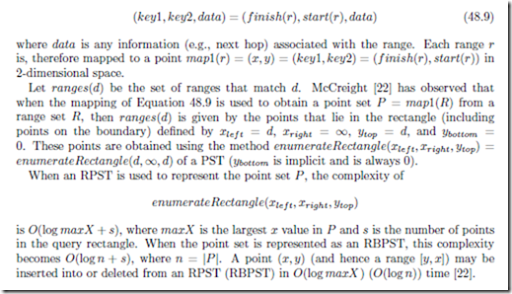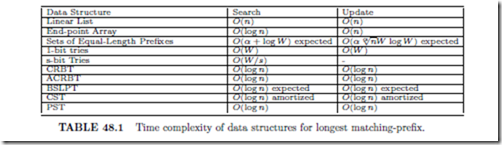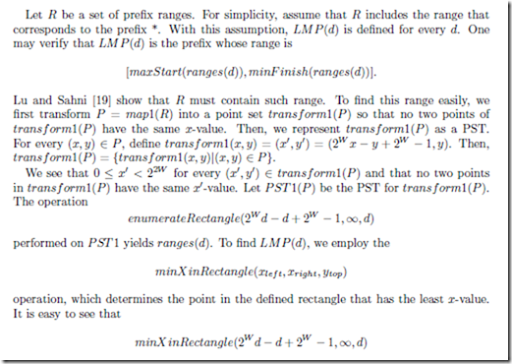performed on PST 1 yields LM P (d).

To insert the preﬁx whose range in [u, v], we insert transf orm1(map1([u, v])) into PST 1. In case this preﬁx is already in PST 1, we simply update the next-hop information for this preﬁx. To delete the preﬁx whose range is [u, v], we delete transf orm1(map1([u, v])) from PST 1. When deleting a preﬁx, we must take care not to delete the preﬁx *. Requests to delete this preﬁx should simply result in setting the next-hop associated with this preﬁx to .

Since, minX inRectangle, insert, and delete each take O(W ) (O(log n)) time when PST 1 is an RPST (RBPST), PST 1 provides a router-table representation in which longest-preﬁx matching, preﬁx insertion, and preﬁx deletion can be done in O(W ) time each when an RPST is used and in O(log n) time each when an RBPST is used.

Tables 48.1 and 48.2 summarize the performance characteristics of various data structures for the longest matching-preﬁx problem.